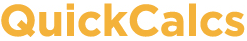1. Select category 2. Choose calculator 3. Enter data 4. View results

## Compare models with Akaike's method and F test

This calculator helps you compare the fit of two models to your data. Enter the goodness-of-fit (sum-of-squares, or weighted sum-of-squares) for each model, as well as the number of data points and the number of parameters for each model. The calculator will compare the models using two methods. First, it uses Akaike's method, which uses information theory to determine the relative likelihood that your data came from each of two possible models. Then it uses the F test (extra sum-of-squares test) to compare the fits using statistical hypothesis testing. Learn more about comparing models in chapters 21–26 of Fitting Models to Biological Data using Linear and Nonlinear Regression.
Number of data points (N):

 Model name Sum-of-squares SS Number of parameters K